# Moving Average

• Updated on

## What is a Moving Average?

A moving average could be defined as a constant period average calculated for each successive period interval. Moving averages are very useful in markets that have a tendency to trend, and they dampen the effect of short-term oscillations.

Moving averages are one of the most widely and conventionally used tools used in technical analysis to smoothen the periodic fluctuations in prices, and they ideally iron out erratic data, making it easier to determine the true underlying trend.

By nature, a moving average is just a number representing a net of certain past averages, i.e., a moving average of 50-day is one number that represents the averages of all prices in the past 50 days.

Many analysts/fund managers prefer to use longer-period moving averages such as 200-day to determine the primary trend in an underlying freely tradable asset.

## Types of Moving Averages

Moving averages could be calculated and simultaneously plotted in a lot many ways on a price chart. Some of the commonly and widely used types of moving average are as below: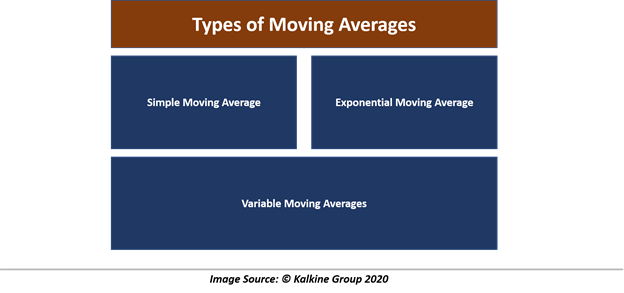## Calculating a Simple Moving Average

Moving averages of prices could be calculated on any type of price data, that is, open, high, low, close. However, the most widely accepted data point for plotting moving averages is the closing price of the day, as many technicians believe that the closing price is the only true price.

They believe so because, the closing price data points often avoid any vast intraday move like high and lows; thus, they are considered as most suitable for smoothing the price fluctuations.10 Day SMA Calculation- Tesla Inc (NASDAQ: TSLA):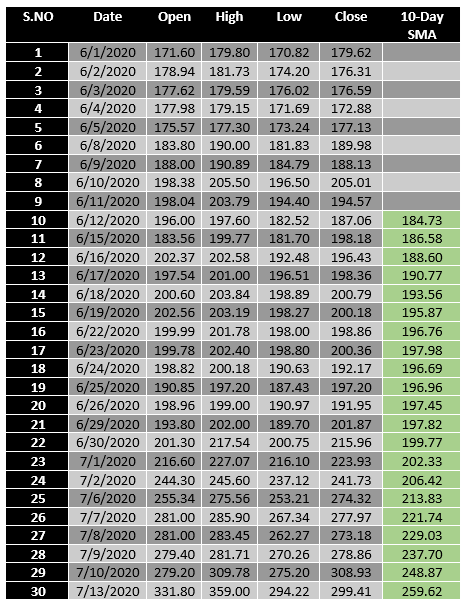A simple moving average is created by summing a set of data and dividing it by the number of data points in the time frame being considered.

For calculation purposes, let us take a look at the recent price data of Tesla Inc (NASDAQ: TSLA), and for simplicity, let’s take the closing price as data point to calculate the 10-day SMA.

To calculate the 10-day SMA, we first sum the closing price of  the first ten days (S.No – 1 to 10) and then divide it by 10 to reach the first 10-day SMA of 184.73. Now, to calculate the moving average on the 11th day we would drop the closing price of the first day from our previous calculation and instead use the 2nd day closing price as the first data point, that is, we would now use the closing price from S.No 2 to 11 to calculate the 10-day SMA of the 11th day (186.58).

## Calculating Exponential Moving Average

Unlike, the simple moving average, exponential moving average does not discard the old data, netting the drop-off effect.

The exponential moving averages use weights to determine the value, and weights for the exponential moving average is calculated in two parts.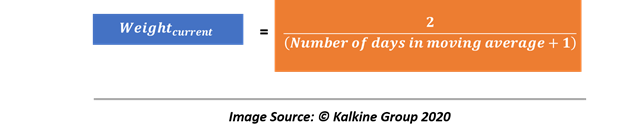For example, if we are calculating the 10-day EMA, we would allocate 2/11 or 18.18% of weight to the closing price of dayt.

Likewise, the remaining weight, i.e., 81.82% would be allocated to the previous day EMA.

The general formula for calculating an EMA on any given day would be as below: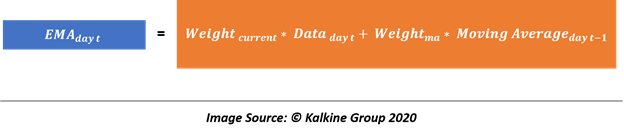So, if we are calculating the 10-day EMA on the 11th day, we would simply allocate 18.18 per cent weight to the closing price of the 11th day, and 81.82% to the previous day moving average.

Furthermore, it should be noticed that the weight of EMA would change if we change the period under consideration. For example, instead of choosing a 10-day EMA, if we were to calculate 15-day EMA, the weight for the current day price would be 12.5% and the remaining 87.5% would be allocated to the previous day moving average.

10 Day EMA Calculation- Tesla Inc (NASDAQ: TSLA):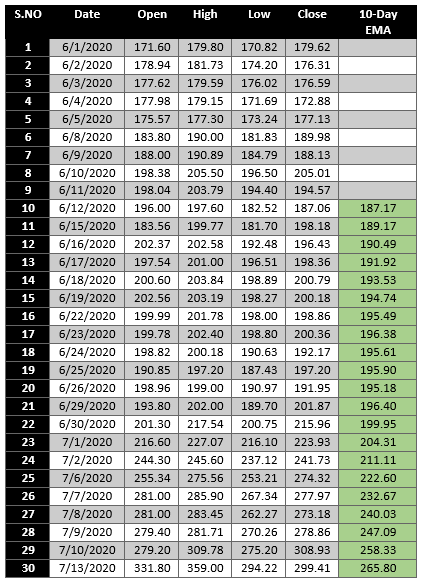## The Drop-Off Effect: SMA Vs EMA

For some analysts, dropping the earlier data could be problematic as the earlier price action might be considered valuable; thus, using the SMA would create an issue as it drops the earlier data in the calculation as discussed above.

A large change in the moving average due to the dropping of the earlier data could generate false trend signals; this effect is typically known as the drop-off effect.

To address the impact of the drop-off effect, a majority of analysts prefer using EMAs as they incorporate the earlier as well as the recent data into the calculation. It does so by simply allocating some weight to the earlier data set; however, EMA also gives higher weightage to the recent price action but does not totally ignore the earlier data.

The use of SMA and EMA depends upon the choice of the analyst and on the purpose of analysis. Sometimes using SMA produces better results and sometimes using EMA produces better results; thus, both the average types go hand-in-hand when it comes to the technical analysis of a trend.

## Variable Moving Averages

• Linearly-weighted moving averages

The linearly-weighted moving average is vast and is typically defined as a type of EMA that allocates linear weights to each data point under consideration. So, for example, if we are calculating a 10-day LWMA, it would multiply the 10th day observation with 10, 9th day observation with 9, and so on.

• Geometric Moving Average

GMA is a simple moving average of percentage change between the two data points.

• Triangular Moving Average

TMA is basically defined as the moving average of a moving average, i.e., doubly smoothed moving average. It primarily constructs a moving average and then calculates the moving average of the previously constructed moving average.

Primarily, trading strategies using moving averages mainly look for a crossover between a short-term moving average and a long-term moving average from below to generate buy signals and vice versa.

When a short-term moving average crosses a long-term moving average from below it is often referred as the golden cross and when a short-term moving average crosses a long-term moving average from above it is often referred as the death cross.

The golden crossover is mainly associated with buy signals and represents a major uptrend while a death cross is mainly associated with sell signals and represents a change in an uptrend. Even fundamental analysts keep a close watch on 200 SMAs or EMAs as it is considered as a good moving average to conclude the strength of bulls and bears from long term perspective.

##### Top ASX Listed Companies

Definition:
We use cookies to ensure that we give you the best experience on our website. If you continue to use this site we will assume that you are happy with it. OK